url = "https://aidworkersecurity.org/incidents/search"

aidworker_df =
aidworker_html %>%
html_nodes(css = "table") %>%
first() %>%
html_table() %>%
as_tibble()

aidworker_df =
aidworker_df %>%
janitor::clean_names() %>%
select(-source, -verified) %>%
rename(year = year_sort_descending) %>%
mutate(intl_org_affected =
case_when(
un != 0 ~ "yes",
ingo != 0 ~ "yes",
icrc_and_ifrc != 0 ~ "yes",
nngo != 0 ~ "no",
other != 0 ~ "yes",
nrcs != 0 ~ "no"),
intl_org_affected = as.factor(intl_org_affected)) %>%
mutate(
latitude = as.numeric(latitude),
longitude = as.numeric(longitude)
) %>%
relocate(id, month, day, year, country, intl_org_affected)

## This is a function that should turn strings with empty spaces into NA
empty_as_na <- function(x){
if("factor" %in% class(x)) x <- as.character(x)
ifelse(as.character(x)!="", x, NA)
}

aidworker_df =
aidworker_df %>% mutate_each(funs(empty_as_na))

aidworker_df =
aidworker_df %>%
filter(country != "Total") %>%
mutate(attack_abr = case_when(
means_of_attack == "Kidnap-killing" ~ "Kidnapping",
means_of_attack == "Kidnapping" ~ "Kidnapping",
means_of_attack == "Body-borne IED" ~ "IED",
means_of_attack == "Vehicle-born IED" ~ "IED",
means_of_attack == "Roadside IED" ~ "IED",
means_of_attack == "Landmine" ~ "Explosives",
means_of_attack == "Shelling" ~ "Explosives",
means_of_attack == "Other Explosives" ~ "Explosives",
means_of_attack == "Aerial bombardment" ~ "Explosives",
means_of_attack == "Rape/sexual assault" ~ "Rape/sexual assault",
means_of_attack == "Complex attack" ~ "Complex attack",
means_of_attack == "Shooting" ~ "Shooting",
means_of_attack == "Unknown" ~ "Unknown",
means_of_attack == "Bodily assault" ~ "Bodily assault"
))

Modern humanitarian action began in 1859 with the founding of the Red Cross movement after activist Henri Dunant witnessed battlefield suffering in Solferino, Italy and envisioned a neutral party that could assist victims of conflict. Aidworkers today follow common core principles of humanity, neutrality, impartiality, and independence to assist any person in need: and as such, should be protected from violence themselves. Unfortunately, the world is becoming more dangerous for those who provide humanitarian assistance.

## Most Dangerous Countries

As the interactive plot below shows, the violence against aid workers is concentrated primarily in a few countries, with a very high number of aid worker victims in Afghanistan.

plot_2 =
aidworker_df %>%
group_by(country) %>%
summarize(tot_attacks = n_distinct(id),
tot_national = sum(total_national_staff),
tot_intl = sum(total_international_staff),
tot_victims = sum(total_victims)) %>%
mutate(rank = min_rank(desc(tot_victims))) %>%
filter(rank < 51) %>%
mutate(country = fct_reorder(country, tot_victims)) %>%
mutate(text_label =
str_c("Total Victims: ", tot_victims, "\nRank: ", rank, "\nInternational Staff: ",
tot_intl, "\nNational Staff: ", tot_national)) %>%
plot_ly(
x = ~country, y = ~tot_victims, text = ~text_label,
type = "scatter", mode = "markers")

layout(plot_2,
margin = list(l=25, r=50, b=100, t=50, pad=0),
title = "Top 50 Most Dangerous Countries for Aid Workers",
xaxis = NULL,
yaxis = list(title = "Total Victims"))

The next two plots look at the top 10 countries for national staff victims and international staff victims: demonstrating the proportion of victims who were wounded, kidnapped, and killed.

danger_countries_df =
aidworker_df %>%
group_by(country) %>%
summarize(tot_affected_per_country = sum(total_national_staff, na.rm = TRUE)) %>%
mutate(rank = min_rank(desc(tot_affected_per_country))) %>%
filter(rank < 11)

aidworker_df %>%
filter(country %in% c("Afghanistan", "Central African Republic", "DR Congo",
"Iraq", "Pakistan", "Somalia", "South Sudan", "Sudan",
"Syrian Arab Republic", "Nigeria")) %>%
group_by(country) %>%
summarize("Killed" = sum(nationals_killed),
"Wounded" = sum(nationals_wounded),
"Kidnapped" = sum(nationals_kidnapped),
tot_natl = sum(total_national_staff)) %>%
pivot_longer(
"Killed":"Kidnapped",
names_to = "Violence_type",
values_to = "Number"
) %>%
mutate(country = fct_reorder(country, tot_natl)) %>%
ggplot(aes(x = as.factor(country), y = Number, fill = Violence_type)) +
geom_bar(stat="identity") +
labs(
title = "Top 10 Most Deadly Countries for Aid Workers (National Staff)",
x = "Country",
y = "National Staff Victims"
) +
viridis::scale_fill_viridis(
name = "Violence Type",
discrete = TRUE
) +
theme(axis.text.x = element_text(angle = -90, vjust = 0.5, hjust=1))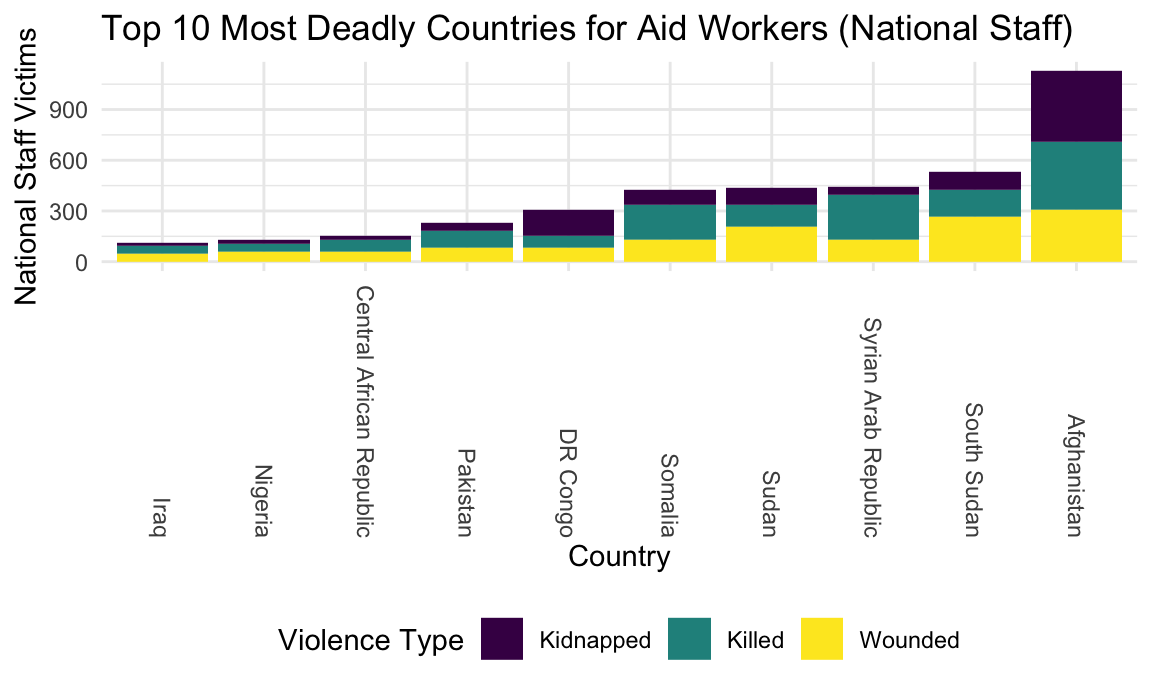In Syria and Somalia, national staff were killed more than they were kidnapped or simply wounded.

danger_countries_df =
aidworker_df %>%
group_by(country) %>%
summarize(tot_affected_per_country = sum(total_international_staff, na.rm = TRUE)) %>%
mutate(rank = min_rank(desc(tot_affected_per_country))) %>%
filter(rank < 11)

aidworker_df %>%
filter(country %in% c("Afghanistan", "Chechnya", "DR Congo",
"Iraq", "Kenya", "Somalia", "South Sudan", "Sudan",
"Syrian Arab Republic", "Yemen", "Libyan Arab Jamahiriya")) %>%
group_by(country) %>%
summarize("Killed" = sum(internationals_killed),
"Wounded" = sum(internationals_wounded),
"Kidnapped" = sum(internationals_kidnapped),
tot_intl = sum(total_international_staff)) %>%
pivot_longer(
"Killed":"Kidnapped",
names_to = "Violence_type",
values_to = "Number"
) %>%
mutate(country = fct_reorder(country, tot_intl)) %>%
ggplot(aes(x = as.factor(country), y = Number, fill = Violence_type)) +
geom_bar(stat="identity") +
labs(
title = "Top 11 Most Deadly Countries for Aid Workers (International Staff)",
x = "Country",
y = "International Staff Victims"
) +
viridis::scale_fill_viridis(
name = "Violence Type",
discrete = TRUE
) +
theme(axis.text.x = element_text(angle = -90, vjust = 0.5, hjust=1))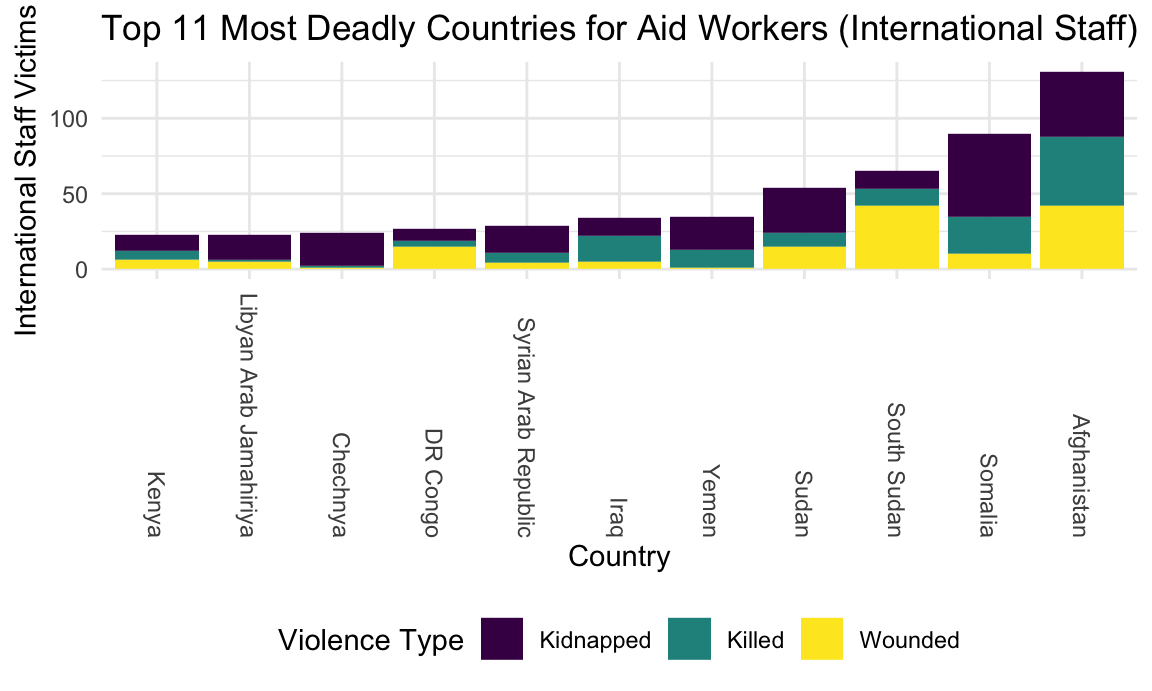Kidnapping comprises a greater proportion of attacks among international staff in many countries than among national staff. Libya and Kenya tied for 10th most international victims.

The table below ranks the top 10 countries by number of attacks and demonstrates the percentage of international and national staff victims who were killed or kidnapped.

aidworker_df %>%
group_by(country) %>%
summarize(attacks = n_distinct(id),
nationals = sum(total_national_staff),
internationals = sum(total_international_staff),
tot_victims = sum(total_victims),
sum_national_kill = sum(nationals_killed),
sum_national_kidnap = sum(nationals_kidnapped),
pct_nationals_killed = (sum_national_kill/nationals)*100,
pct_nationals_kidnapped = (sum_national_kidnap/nationals)*100,
sum_intl_kill = sum(internationals_killed),
sum_intl_kidnap = sum(internationals_kidnapped),
pct_intl_killed = (sum_intl_kill/internationals)*100,
pct_intl_kidnapped = (sum_intl_kidnap/internationals)*100) %>%
mutate(rank = min_rank(desc(attacks))) %>%
filter(rank < 11) %>%
arrange(rank) %>%
select(country, rank, attacks, nationals, pct_nationals_killed,
pct_nationals_kidnapped, internationals, pct_intl_killed, pct_intl_kidnapped) %>%
knitr::kable()
country rank attacks nationals pct_nationals_killed pct_nationals_kidnapped internationals pct_intl_killed pct_intl_kidnapped
Afghanistan 1 561 1127 36.11358 36.82343 131 35.11450 32.82443
South Sudan 2 366 534 30.52434 20.03745 65 16.92308 18.46154
Syrian Arab Republic 3 290 441 60.31746 10.43084 29 24.13793 62.06897
Somalia 4 276 426 48.35681 21.12676 90 27.77778 61.11111
Sudan 5 266 437 28.60412 23.56979 54 16.66667 55.55556
DR Congo 6 169 308 23.05195 49.67532 27 14.81481 29.62963
Pakistan 7 108 232 42.24138 22.41379 21 33.33333 14.28571
Central African Republic 8 102 151 45.69536 15.89404 16 18.75000 31.25000
Yemen 9 73 112 28.57143 35.71429 35 34.28571 62.85714
Iraq 10 67 112 42.85714 16.07143 34 50.00000 35.29412

Finally, this plot shows victims of the top 5 most dangerous countries over time. Context for the spike in attacks in Afghanistan in 2013 is discussed in the accompanying “Zoom In” tab. A rise in attacks in Syria corresponds to the start of the Syrian Civil War in 2011.

danger_countries_df =
aidworker_df %>%
group_by(country) %>%
summarize(tot_affected_per_country = sum(total_victims, na.rm = TRUE)) %>%
mutate(rank = min_rank(desc(tot_affected_per_country))) %>%
filter(rank < 11)

aidworker_df %>%
filter(country %in% c("Afghanistan", "Somalia", "South Sudan", "Sudan",
"Syrian Arab Republic")) %>%
group_by(country, year) %>%
summarize(tot_victims = sum(total_victims)) %>%
ggplot(aes(x = year, y = tot_victims, color = country)) +
geom_line() +
labs(
title = "Top 5 Most Dangerous Countries Over Time",
x = "Year",
y = "Total Aidworker Victims"
) +
theme(legend.text=element_text(size=7))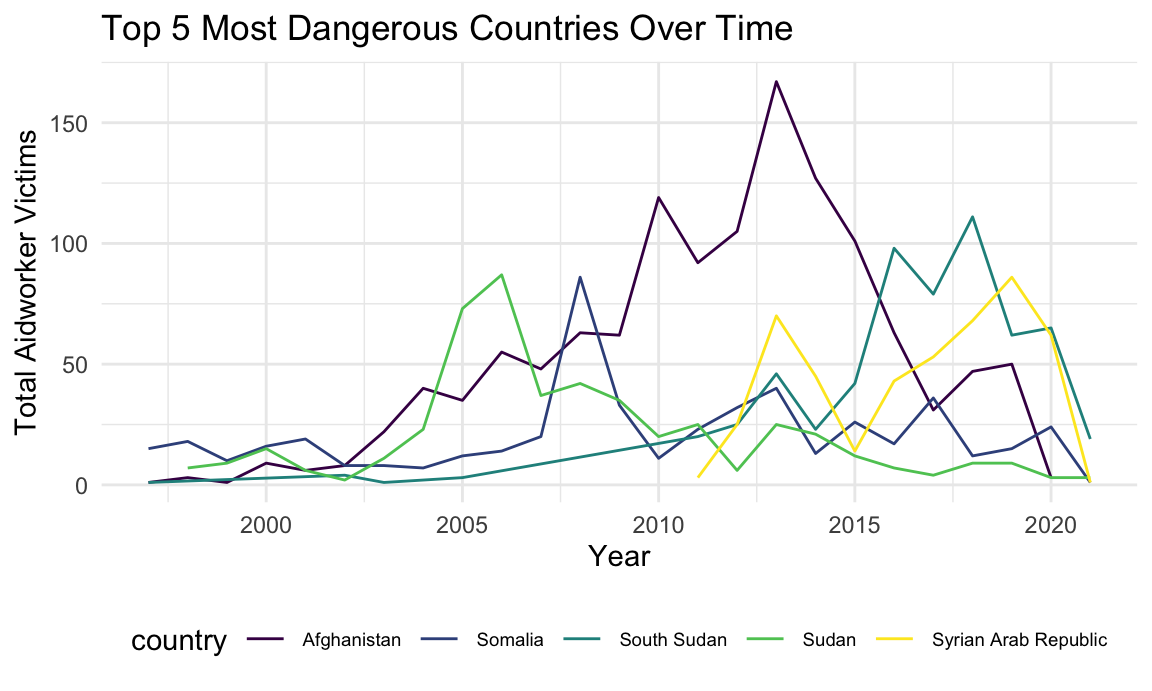## Attack Contexts

As we see below, most aidworker attacks globally occur during transit, on roadways. The large portion of ‘unknown’ attack locations and attack types is indicative of challenges in collecting comprehensive data during humanitarian emergencies.

aidworker_df %>%
group_by(location, attack_abr) %>%
summarize(attacks = n_distinct(id)) %>%
mutate(location = as.factor(location),
location = fct_reorder(location, attacks)) %>%
ggplot(aes(x = location, y = attacks, fill = attack_abr)) +
geom_bar(stat="identity") +
coord_flip() +
labs(title = "Global view: Attack Locations and Types of Attacks",
x = "Location of attack",
y = "Number of attacks") +
viridis::scale_fill_viridis(
name = "Attack type",
discrete = TRUE
) +
theme(legend.text=element_text(size=7))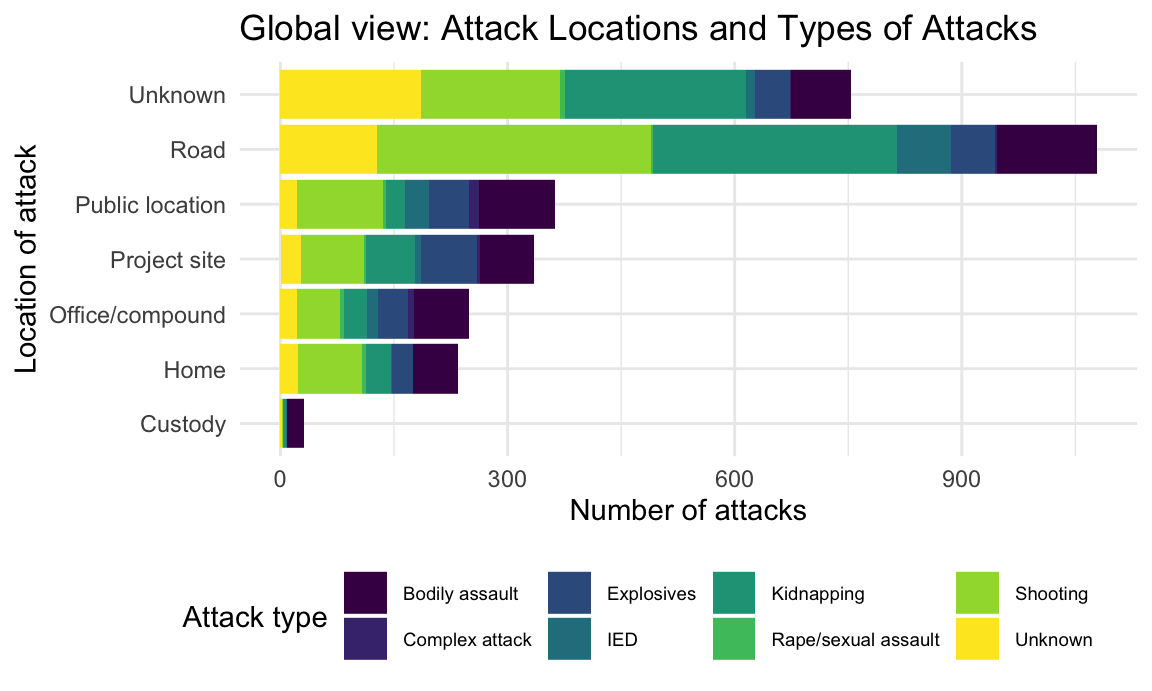The 5 most dangerous countries are shown below with type and contexts of attacks. Here, attack type refers to the means of violence, while attack context refers to the broader context of the incident.

danger_countries_df =
aidworker_df %>%
group_by(country) %>%
summarize(tot_attacks = sum((n_distinct(id)), na.rm = TRUE)) %>%
mutate(rank = min_rank(desc(tot_attacks))) %>%
filter(rank < 6)

aidworker_df %>%
filter(country %in% c("Afghanistan", "Somalia", "South Sudan", "Sudan",
"Syrian Arab Republic")) %>%
group_by(country, attack_abr) %>%
summarize(attacks = n_distinct(id)) %>%
mutate(country = as.factor(country),
location = fct_reorder(country, attacks)) %>%
ggplot(aes(x = country, y = attacks, fill = attack_abr)) +
geom_bar(stat="identity") +
labs(title = "Most Dangerous Countries: Attack Types",
x = "Country",
y = "Number of Attacks") +
theme(axis.text.x = element_text(angle = -90, vjust = 0.5, hjust=1)) +
theme(legend.text=element_text(size=7)) +
viridis::scale_fill_viridis(
name = "Attack type",
discrete = TRUE
)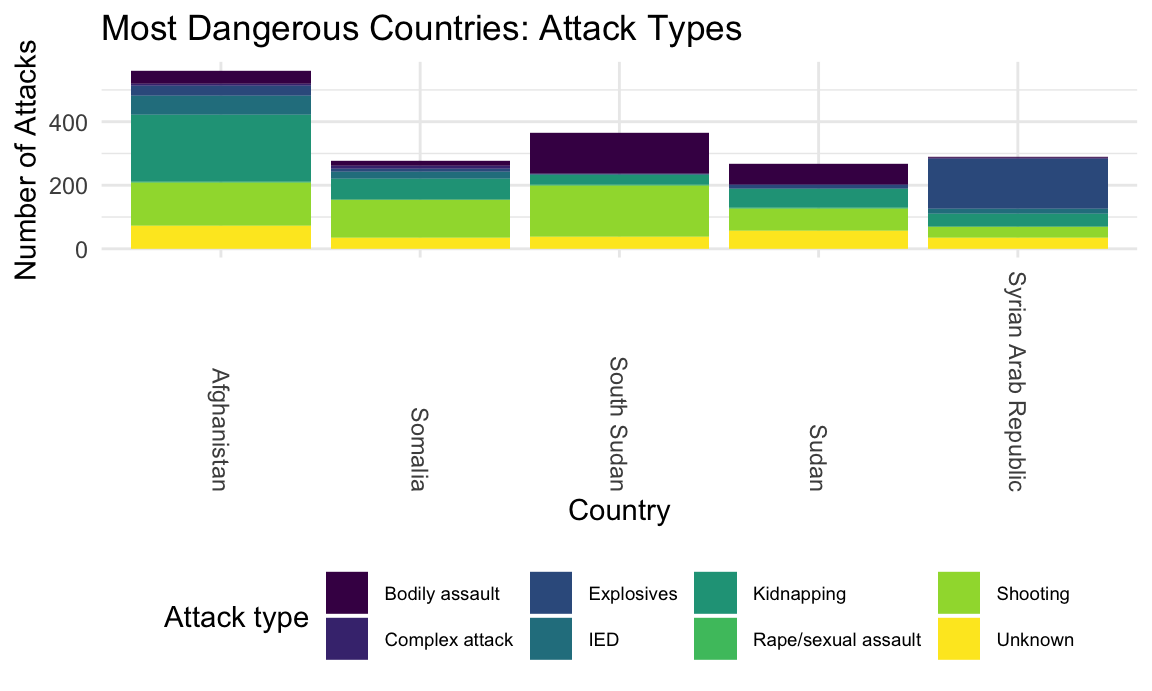aidworker_df %>%
filter(country %in% c("Afghanistan", "Somalia", "South Sudan", "Sudan",
"Syrian Arab Republic")) %>%
group_by(country, attack_context) %>%
summarize(attacks = n_distinct(id)) %>%
mutate(country = as.factor(country),
location = fct_reorder(country, attacks)) %>%
ggplot(aes(x = country, y = attacks, fill = attack_context)) +
geom_bar(stat="identity") +
labs(title = "Most Dangerous Countries: Attack Contexts",
x = "Country",
y = "Number of Attacks") +
theme(axis.text.x = element_text(angle = -90, vjust = 0.5, hjust=1)) +
theme(legend.text=element_text(size=7)) +
viridis::scale_fill_viridis(
name = "Attack Context",
discrete = TRUE
)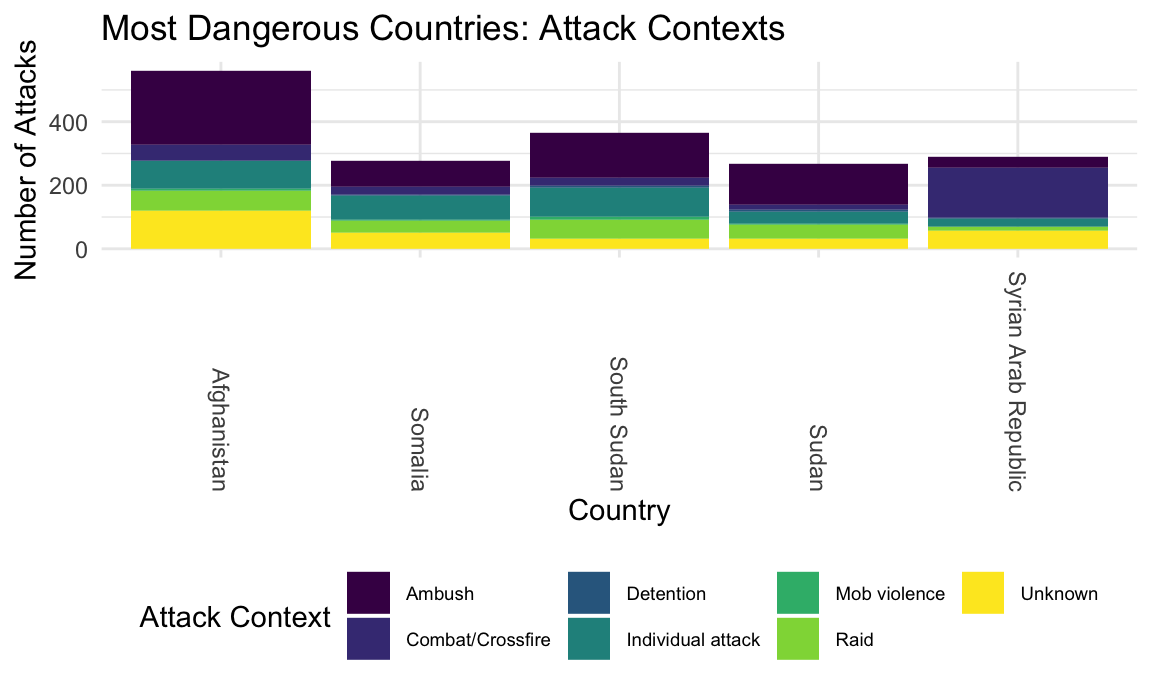Afghanistan experienced the greatest proportion of attacks by kidnapping or IEDs, while bodily assault was most common in South Sudan, and explosives (including all bombing, shelling, and aerial bombardment) were most common in Syria.

Ambushing, individual attacks, and raids were common in all danger countries. Aid workers in Syria experienced a large number of attacks by combat or crossfire: although the AWSD notes this does not exclude intentional targeting of humanitarian locations by combatants. Intentional targeting of health facilities in particular by Russian and Syrian airstrikes has been well documented. (via The Century Foundation)

Finally, the interactive plot below depicts the gender gap in aid worker victims for the most dangerous countries. Men outnumber women for all attack types other than sexual assault. Bubble size corresponds to the degree of gap, with a bubble for each attack type in each country.

plot_gender =
aidworker_df %>%
filter(country %in% c("Afghanistan", "Somalia", "South Sudan", "Sudan",
"Syrian Arab Republic")) %>%
group_by(country, attack_abr) %>%
summarize(total_women = sum(gender_female),
total_men = sum(gender_male),
gap = total_men - total_women,
tot_victims = sum(total_victims)) %>%
mutate(text_label =
str_c("Country: ", country, "\nAttack Type: ", attack_abr)) %>%
plot_ly(
x = ~total_men, y = ~total_women, text = ~text_label, color = ~country, size = ~gap,
type = "scatter", mode = "markers",  colors = "viridis",
sizes = c(50, 700), marker = list(opacity = 0.7))

layout(plot_gender, title = "Dangerous Country Gender Gap by Attack Type", xaxis = list(title = "Number of Men Attacked"), yaxis = list(title = "Number of Women Attacked"))